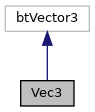SuperTuxKart
Searching...
No Matches
Vec3 Class Reference

A wrapper around bullets btVector3 to include conventient conversion functions (e.g. More...

`#include <vec3.hpp>`

Inheritance diagram for Vec3:[legend]

## Public Member Functions

Vec3 (const core::vector3df &v)
Convert an irrlicht vector3df into the internal (bullet) format.

Vec3 (const btVector3 &a)
Initialises a vector from a btVector3 (or a Vec3).

Vec3 ()
Empty constructor.

Vec3 (float x, float y, float z)
Creates a 3d vector from three scalars.

Vec3 (float x, float y, float z, float w)
Creates a 3d vector from three scalars.

Vec3 (float x)
Initialises a 3d vector from one scalar value, which is used to initialise all components.

Vec3 (float heading, const Vec3 &normal)
Sets the heading, and computes pitch and roll dependent on the normal it is displayed on.

void setHPR (const btQuaternion &q)
Sets the heading, pitch, roll of this vector that is used to store a rotation from a quaternion.

const float & operator[] (int n) const
Returns a reference to the n-th element (x=0, y=1, z=2, w=3).

float & operator[] (int n)
Returns a reference to the n-th element (x=0, y=1, z=2, w=3).

Returns the heading of a vector that is used to store a rotation.

const float getPitch () const
Returns the pitch of a vector that is used to store a rotation.

const float getRoll () const
Returns the roll of a vector that is used to store a rotation.

const float getW () const
Returns the W component (bullet vectors contain 4 elements, the last element is usually unused).

Sets the heading of a vector that is used to store a rotation.

const void setPitch (float f)
Sets the pitch of a vector that is used to store a rotation.

const void setRoll (float f)
Sets the roll of a vector that is used to store a rotation.

const core::vector3df & toIrrVector () const
Converts a vec3 into an irrlicht vector (which is a simple type cast).

const core::vector3df toIrrHPR () const
Converts a bullet HPR value into an irrlicht HPR value.

const core::vector2df toIrrVector2d () const
Returns the X and Z component as an irrlicht 2d vector.

Converts degree values stored in this vec3 to radians.

Vec3operator= (const btVector3 &a)
Sets this = a.

Vec3operator= (const btQuaternion &q)
Sets the rotation given by the quaternion as HPR vector.

bool operator== (const Vec3 &other) const
Operator== of btQuadWord also compares m_floats, which is not useful (and wrong in certain circumstances).

bool operator!= (const Vec3 &other) const
Operator!= of btQuadWord also compares m_floats, which is not useful (and wrong in certain circumstances).

Vec3 operator- (const Vec3 &v1) const
Computes this = this - v1.

Vec3 operator- (const btVector3 v1) const
Computes this = this - v1.

float length2_2d () const
Helper functions to treat this vec3 as a 2d vector.

float length_2d () const
Returns the length of this vector in the plane, i.e.

void max (const Vec3 &a)
Sets this = max(this, a) componentwise.

void min (const Vec3 &a)
Sets this = min(this, a) componentwise.

float sideOfLine2D (const Vec3 &start, const Vec3 &end) const
Determines which side of a line this point is.

float sideofPlane (const Vec3 &x1, const Vec3 &x2, const Vec3 &x3) const

## Private Member Functions

void setPitchRoll (const Vec3 &normal)
Sets the pitch and the roll of this vector to follow the normal given.

## Detailed Description

A wrapper around bullets btVector3 to include conventient conversion functions (e.g.

between btVector3 and the graphics specific 3d vector).

## ◆ Vec3() [1/2]

 Vec3::Vec3 ( const core::vector3df & v )
inline

Convert an irrlicht vector3df into the internal (bullet) format.

Irrlicht's and STK's axis are different (STK: Z up, irrlicht: Y up). We might want to change this as well, makes it easier to work with bullet and irrlicht together, without having to swap indices (bullet can handle any axis ordering). Note that toIrrVector swaps the axis as well (so a vector3df can be stored in and restored from a vec3).

## ◆ Vec3() [2/2]

 Vec3::Vec3 ( float heading, const Vec3 & normal )
inline

Sets the heading, and computes pitch and roll dependent on the normal it is displayed on.

Parameters
 heading The heading to set. normal The normal from which pitch and roll should be computed.

## ◆ length2_2d()

 float Vec3::length2_2d ( ) const
inline

Helper functions to treat this vec3 as a 2d vector.

This returns the square of the length of the first 2 dimensions.

## ◆ length_2d()

 float Vec3::length_2d ( ) const
inline

Returns the length of this vector in the plane, i.e.

the vector is used as a 2d vector. Returns the length of the vector using only the x/z coordinates.

## ◆ max()

 void Vec3::max ( const Vec3 & a )
inline

Sets this = max(this, a) componentwise.

Parameters
 Vector to compare with.

## ◆ min()

 void Vec3::min ( const Vec3 & a )
inline

Sets this = min(this, a) componentwise.

Parameters
 a Vector to compare with.

## ◆ operator-()

 Vec3 Vec3::operator- ( const btVector3 v1 ) const
inline

Computes this = this - v1.

On VS this special version is needed, since otherwise Vec3-btVector3 is ont unique (could be cast to btVector3-btVector3, or convert btVector3 to Vec3()).

## ◆ setPitchRoll()

 void Vec3::setPitchRoll ( const Vec3 & normal )
private

Sets the pitch and the roll of this vector to follow the normal given.

The heading is taken from this vector.

Parameters
 normal The normal vector to which pitch and roll should be aligned.

## ◆ sideOfLine2D()

 float Vec3::sideOfLine2D ( const Vec3 & start, const Vec3 & end ) const
inline

Determines which side of a line this point is.

This is using a 2d projection (into the X-Z plane).

Parameters
 start The start point of the line. end The end point of the line.
Returns
>0 Point is to the left side; <0 if it's on the right.

The documentation for this class was generated from the following files: